﻿ 涂层式裂纹监测系统中基体裂纹穿越行为研究
«上一篇文章快速检索 高级检索

 力学学报2015, Vol. 47Issue (5): 772-778  DOI: 10.6052/0459-1879-15-1420

### 引用本文 [复制中英文]

[复制中文]
Sun Yang, Wang Biao, Wang Qiaoyun, Liu Mabao. STUDY OF THE SUBSTRATE CRACK PENETRATION MECHANISMS IN CRACK-DETECTED COATING SYSTEM[J]. Chinese Journal of Ship Research, 2015, 47(5): 772-778. DOI: 10.6052/0459-1879-15-142.
[复制英文]

### 文章历史

2015-04-21收稿
2015-06-29录用
2015–07–01网络版发表

1. 西安交通大学机械结构强度与振动国家重点实验室, 西安710049;
2. 西安航空学院, 西安710077

1 分 析 1.1 问题描述

 $\left. {\begin{array}{*{20}{l}} \begin{array}{l} {\alpha _i} = \frac{{{E_{i + 1}}(1 - \nu _i^2) - {E_i}(1 - \nu _{i + 1}^2)}}{{{E_{i + 1}}(1 - \nu _i^2) + {E_i}(1 - \nu _{i + 1}^2)}}\\ 2{\beta _i} = \frac{{{E_{i + 1}}(1 + {\nu _i})(1 - 2{\nu _i}) - {E_i}(1 + {\nu _{i + 1}})(1 - 2{\nu _{i + 1}})}}{{{E_{i + 1}}(1 - \nu _i^2) + {E_i}(1 - \nu _{i + 1}^2)}} \end{array} \end{array}} \right\}$ (1)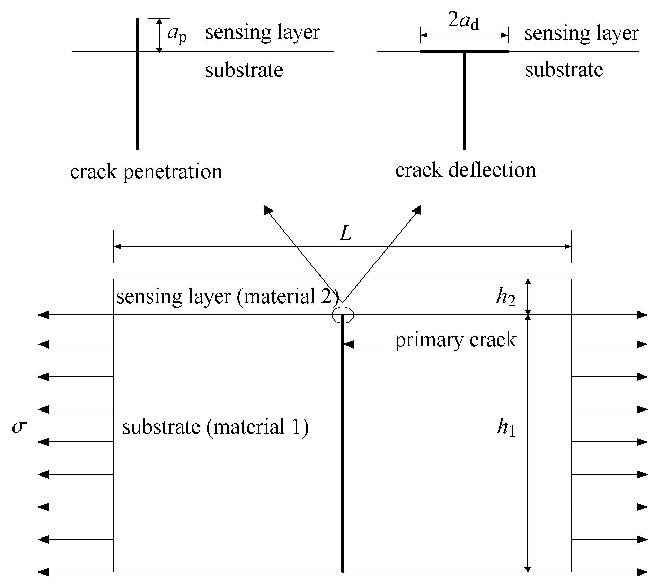图 1 两相模型中智能涂层基体裂纹的穿越和偏转 Fig. 1 The substrate crack penetration and deflection for the two-phase model of the smart coating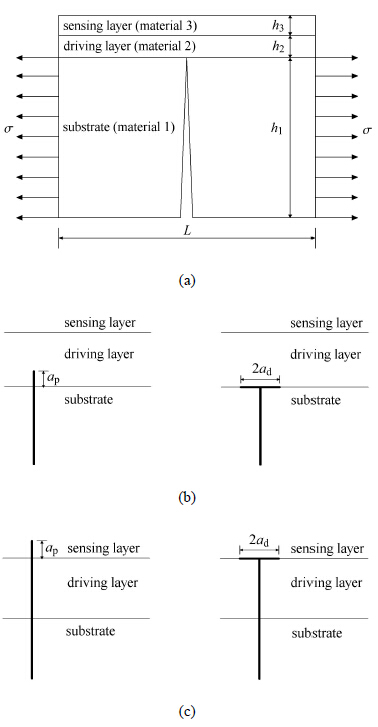图 2 三相模型中智能涂层基体裂纹的穿越和偏转 Fig. 2 The substrate crack penetration and deflection for the three-phase model of the smart coating

 $\frac{{{\Gamma _{\rm{d}}}}}{{{\Gamma _{\rm{p}}}}} > K = \frac{{{G_{\rm{d}}}}}{{{G_{\rm{p}}}}} = f(\alpha ,\beta ){(\frac{{{a_{\rm{d}}}}}{{{a_{\rm{p}}}}})^{1 - 2\lambda }}$ (2)

 $\cos \lambda \pi = \frac{{2(\beta - \alpha )}}{{1 + \beta }}{(1 - \lambda )^2} + \frac{{\alpha + {\beta ^2}}}{{1 - {\beta ^2}}}$ (3)

 $\frac{{{G_{\rm{d}}}}}{{{G_{\rm{p}}}}} < \frac{{{\Gamma _{{\rm{d1}}}}}}{{{\Gamma _{{\rm{p2}}}}}}$ (4)

 $\frac{{{G_{\rm{d}}}}}{{{G_{\rm{p}}}}} < \frac{{_{\Gamma {\rm{d2}}}}}{{{\Gamma _{{\rm{p3}}}}}}$ (5)

1.2 数值分析

 $\left. {\begin{array}{*{20}{l}} \begin{array}{l} \frac{{{E_{i + 1}}}}{{{E_i}}} = \frac{{(1 - \nu _{i + 1}^2)(1 + {\alpha _i})}}{{(1 - \nu _i^2)(1 - {\alpha _i})}}\\ {\nu _i} = \frac{{2{\alpha _i} - 3{\nu _{i + 1}}{\alpha _i} + {\nu _{i + 1}}}}{{1 + 3{\alpha _i} - 4{\nu _{i + 1}}{\alpha _i}}} \end{array} \end{array}} \right\}$ (6)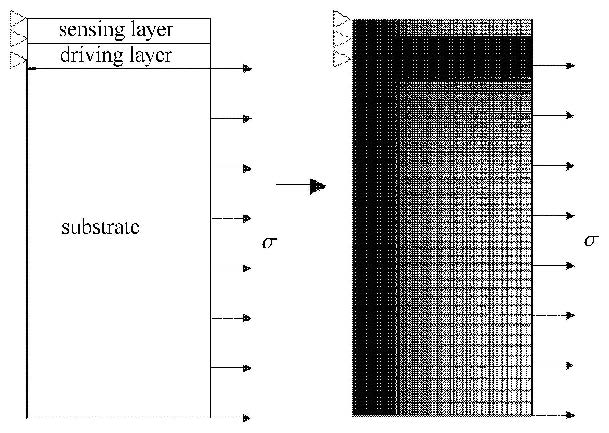图 3 三相模型中智能涂层基体裂纹穿越偏转有限元模型 Fig. 3 The finite element model of the substrate crack deflection penetration for three-phase model of the smart coating
2 数值结果和讨论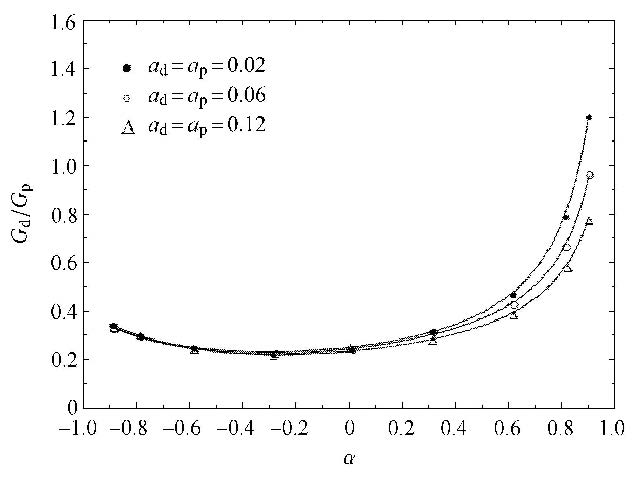图 4 $G_{\rm d}/G_{\rm p}$随不同的$a_{\rm d}=a_{\rm p}$时的变化结果 Fig. 4 Result between the values of $G_{\rm d}/G_{\rm p}$ for various propagation length $a_{\rm d}=a_{\rm p}$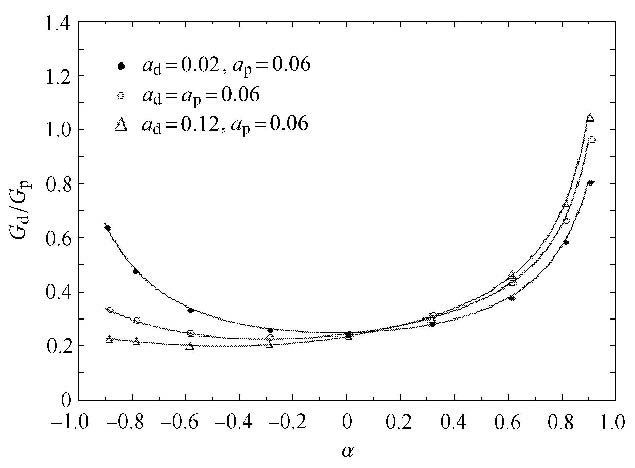图 5 $G_{\rm d}/G_{\rm p}$随不同的$a_{\rm d} \ne a_{\rm p}$时的变化结果 Fig. 5 Result between the values of $G_{\rm d}/G_{\rm p}$ for various propagation length $a_{\rm d} \ne a_{\rm p}$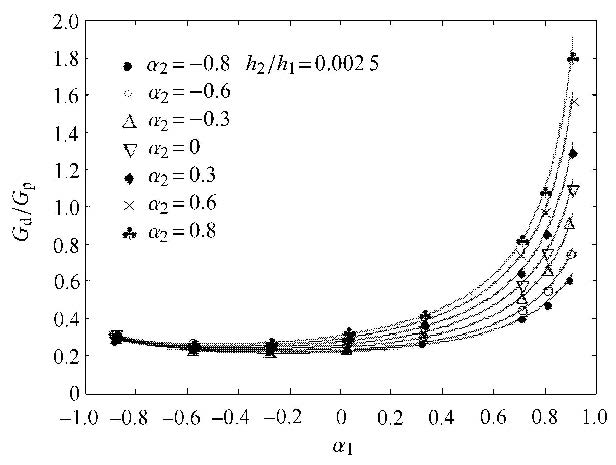图 6 $h_{2}/h_{1}= 0.002\,5$时，$G_{\rm d}/G_{\rm p}$随 $\alpha_{1}$和$\alpha_{2}$的变化结果 Fig. 6 Result between the values of $G_{\rm d}/G_{\rm p}$ and $\alpha_{1}$, $\alpha_{2}$ for $h_{2}/h_{1}= 0.002\,5$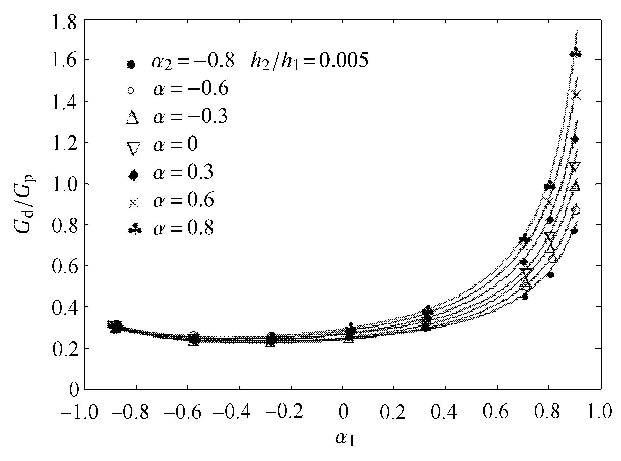图 7 $h_{2}/h_{1}= 0.005$时，$G_{\rm d}/G_{\rm p}$随$\alpha _{1}$和$\alpha_{2}$的变化结果 Fig. 7 Result between the values of $G_{\rm d}/G_{\rm p}$ and $\alpha_{1}$, $\alpha _{2}$ for $h_{2}/h_{1}= 0.005$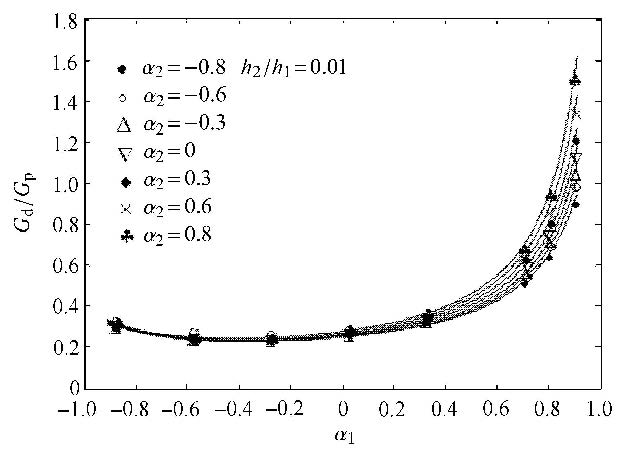图 8 $h_{2}/h_{1}= 0.01$时，$G_{\rm d}/G_{\rm p}$随$\alpha_{1}$和$\alpha_{2}$的变化结果 Fig. 8 Result between the values of $G_{\rm d}/G_{\rm p}$ and $\alpha_{1}$, $\alpha _{2}$ for $h_{2}/h_{1}= 0.01$

3 实验验证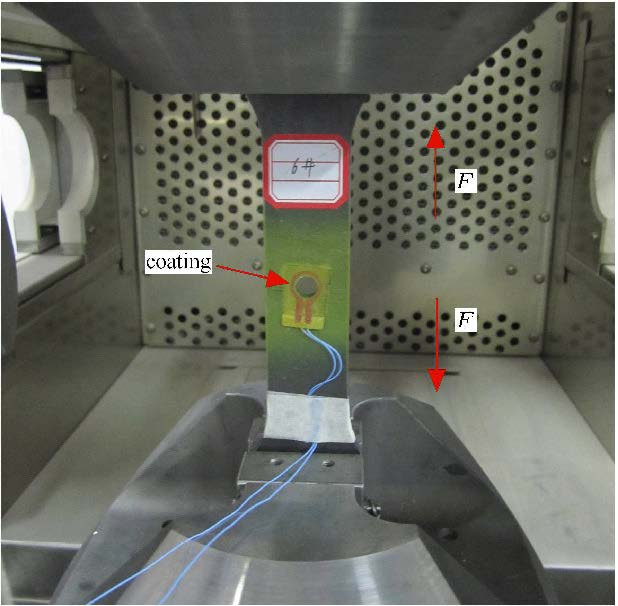图 9 单轴拉伸测试 Fig. 9 Uniaxial tension tests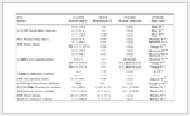表 1 基体、驱动层、传感层的力学性能 Table 1 Mechanical properties of substrate, driving layer and sensing layer图 10 穿越裂纹及断裂面 Fig. 10 The penetrated crack and the fracture surfaces

4 结 论

(1) 对于两相模型，当 $\alpha >0$，随着$a_{\rm d}=a_{\rm p}$的增加，能量释放率之比$K=G_{\rm d}/G_{\rm p}$逐渐降低. 然而，当 $\alpha < 0$时，$K$值对假定的裂纹扩展长度不是很敏感. 对于$a_{\rm d} \ne a_{\rm p}$的情形，数值结果表明，当 $\alpha < 0$时，与$a_{\rm d}=a_{\rm p}$时的$K$值相比，$a_{\rm d}>a_{\rm p}$时产生的$K$值较小，这将有利于裂纹的穿越，而当 $\alpha >0$时，将会产生较大的$K$.

(2) 对于三相模型，数值分析表明$K$值不仅依赖于弹性错配参数 $\alpha_{i}$ ($i =1$, 2)，而且依赖于驱动层的相对厚度. 进一步的数值分析表明$K$对大的正 $\alpha_{1}$有较强的依赖性，而对大的负$\alpha_{1}$却不太敏感. 另外，较大的$\alpha_{2}$通常产生较大的$K$值，这将降低裂纹穿越的能力. 数值结果亦表明，对于$\alpha_{2}>0$，驱动层越厚，$K$值越小，这种情况有利于裂纹的穿越. 而对于 $\alpha_{2} < 0$,驱动层越厚，$K$值越大，这种情况不利于裂纹的穿越. 另外，随着驱动层厚度的增加，$\alpha_{2}$对$K$值的影响逐渐降低.

(3) 实验结果表明基体裂纹能够相继穿越进入驱动层和传感层，说明了数值分析的正确性. 合理选择驱动层与传感层的弹性模量及厚度对智能涂层传感器的优化设计具有非常重要的作用.

  袁慎芳,梁栋,高宁等. 基于结构健康监测系统的桥梁数据异常诊断研究. 电子科技大学学报,2013 42(1):69-74 (Yuan Shen-fang, Liang Dong, Gao Ning, et al. The bridge data diagnosis research based on structural health monitoring system. Journal of University of Electronic Science and Technology of China, 2013, 42(1): 69-74 (in Chinese))  杨建良,郭照华,向清等. 基于神经网络与光纤传感阵列的结构状态监测方法. 力学学报,1998, 30 (2):213-219 (Yang Jianliang, Guo Zhaohua, Xiang Qing, et al. Structural state detec-tion with fiberoptic sensing array and neural network signal processing techniques. Acta Mechanica Sinica, 1998, 30 (2):213-219 (in Chinese))  Ignatovich SR, Menou A, Karuskevich MV, et al. Fatigue damage and sensor development for aircraft structural health monitoring. Theoretical and Applied Fracture Mechanics, 2013, 65: 23-27  孙侠生,肖迎春. 飞机结构健康监测技术的机遇与挑战. 航空学报, 2014,35(12):3199-3212 (Sun Xiasheng, Xiao Yingchen. Opportu-nities and challenges of aircraft SHM. Acta Aeronoutica et Astronautica Sinica, 2014, 35(12): 3199-3212(in Chinese))  Hu F, Liu MB, Gao H, et al. Flaw-detected coating sensors applied in aircraft R&M. In: Proc. of 55th Annual Reliability & Maintainability Symposium. New York: IEEE, 2009: 95-99  Liu MB, Sun Y, Wang Z, et al. A validation study for a shm technology under operational environment. In: Proc. of 60th Annual Reliability and Maintainability Symposium (RAMS).New York: IEEE, 2014  Sun Y, Liu MB. Analysis of the crack penetration/deflection at the inter-faces in the intelligent coating system utilizing virtual crack closure tech-nique. Engineering Fracture Mechanics, 2015, 133: 152-162  Cook J, Gordon JE, Evans CC, et al. A mechanism for the control of crack propagation in all-brittle systems. Proceedings of the Royal Society of London A, 1964, 282(1391): 508-520  Gupta V, Suo Z, Argon AS. Crack deflection at an interface between two orthotropic media. Journal of Applied Mechanics, 1992, 59(2): S79-87  He MY, Evans AG, Hutchinson JW. Crack deflection at an interface between dissimilar elastic material: Role of residual stresses. International Journal of Solids and Structure, 1994, 31(24): 3443-3455  Liu YF, Tanaka Y, Masuda C. Debonding mechanisms in the presence of an interphase in composites. Acta Materialia, 1998, 46(15): 5237-5247  Ahn BK, Curtin WA, Parthasarathy TA, et al. Criteria for crack deflec-tion/penetration for fiber-reinforced ceramic matrix composites. Composites Science and Technology, 1998, 58(11): 1775-1784  Lee W, Yoo YH, Shin H. Reconsideration of crack deflection at planar interfaces in layered systems. Composites Science and Technology, 2004, 64(15): 2415-2423  Huang B, Zhao MH, Gao CF, et al. Phase angle in indentation-induced delamination with buckling. Scripta Materialia, 2004, 50: 607-611  Jia HK, Wang SB, Li LA, et al. Application of optical 3D measurement on thin film buckling to estimate interfacial toughness. Optics and Lasers in Engineering, 2014, 54: 263-268  Dundurs J. Edge-bonded dissimilar orthogonal elastic wedges. Journal of Applied Mechanics, 1969, 36(3): 650-652  胡峰. 信息智能涂层及其在飞机结构健康监测技术中的应用研究. [硕士论文]. 西安:西安交通大学,2007 (Hu Feng. Intelligent coating and its application in aircraft structural health monitoring. [Master Thesis]. Xi'an: Xi'an Jiaotong University, 2007 (in Chinese))
STUDY OF THE SUBSTRATE CRACK PENETRATION MECHANISMS IN CRACK-DETECTED COATING SYSTEM
Sun Yang, Wang Biao, Wang Qiaoyun, Liu Mabao1. State Key Laboratory for Strength and Vibration of Mechanical Structures, Xi'an Jiaotong University, Xi'an 710049, China;
2. Xi'an Aeronautical University, Xi'an 710077, China
Fund: The project was supported by the National Natural Science Foundation of China (51175404) and Xi’an Lotus Lake District funded projects (K2013-026).
Abstract: The two-phase model and three-phase model of crack penetration/deflection at the interfaces in the crack-detected smart coating system were established utilizing the energy-based criterion. The effects of the relative crack growth length, the elastic mismatch parameters and the thickness of the interface layer on the ratio of energy release rates for the penetrated and deflected cracks were studied by the finite element method. When the substrate crack reaches an interface between substrate and driving layer, the results show that the ratio of energy release rate not only has a strong dependence on elastic mismatch between driving layer and substrate, but elastic mismatch between sensing layer and driving layer for the thinner driving layer as well. Moreover, with increase of the thickness of the driving layer, the dependence on elastic mismatch between sensing layer and driving layer decreases gradually. Compared with the experiment results, the numerical results can interpret the interface behavior of substrate crack and can be used for the optimization design of the crack-detected coating sensors.
Key words: smart coating    crack penetration/deflection    energy criterion    finite element method    interface